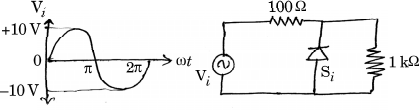MORE IN Basic Electronics Engineering (BXE)
SPPU First Year Engineering (Semester 1)
Basic Electronics Engineering (BXE)
December 2015
Total marks: --
Total time: --
INSTRUCTIONS
(1) Assume appropriate data and state your reasons
(2) Marks are given to the right of every question
(3) Draw neat diagrams wherever necessary

Solve any one question from Q1 and Q2
1 (a) Compare Half Wave and Full Wave Rectifier.
6 M
1 (b) Explain operation of n-channel enhancement type MOSFET with its characteristics.
6 M

2 (a) Determine output waveform for the circuit shown in figure:6 M
2 (b) Draw the output characteristics of BJT in CE configuration. Indicate all the three regions of operation on it. Explain the operation of BJT as a switch.
6 M

Solve any one question from Q3 and Q4
3 (b) Draw the circuit diagram and write output equation for:
i) Non-inverting summer with three inputs
ii) Ideal Differentiator.
6 M
3 (b) Compare Microprocessor and Microcontroller.
4 M
3 (c) Prove the following using De Morgan's Theorem: $$\overline {(A+B) \cdot (C+D)} = (\overline A \cdot \overline B) + (\overline C \cdot \overline D)$$.
2 M

4 (a) For inverting amplifier using op-amp if Rf=100 kΩ, R1=10 kΩ, VCC= ±10 V, Vi=2V d.c:
i) Calculate output voltage
ii) Is the result in part (i) is practically possible? Justify.
6 M
4 (b) How to implement full adder using 2 half adder and logic gates? Explain.
6 M

Solve any one question from Q5 and Q6
5 (a) Draw Block diagram of electronic weighing machine and explain its operation.
6 M
5 (b) Explain the construction of DIAC. Draw and explain its characteristics.
7 M

6 (a) Explain digital thermometer with block diagram.
6 M
6 (b) Define the following terms for SCR:
(i) Holding current
ii) Latching current
iii) Forward breakover voltage
iv) Reverse breakover voltage
v) Turn ON time for SCR.
5 M
6 (c) List applications of SCR.
2 M

Solve any one question from Q7 and Q8
7 (a) What is need of modulation? Explain frequency modulation in detail.
7 M
7 (b) Draw and explain block diagram of mobile communication system.
6 M

8 (a) Draw AM waveform for:
i) Modulation index = 1
ii) Modulation index > 1
iii) Modulation index < 1.
3 M
8 (b) Write short notes on:
i) Twisted Pair Cable
ii) Fiber Optic Cable.
6 M
8 (c) Compare amplitude modulation and frequency modulation.
4 M

More question papers from Basic Electronics Engineering (BXE)